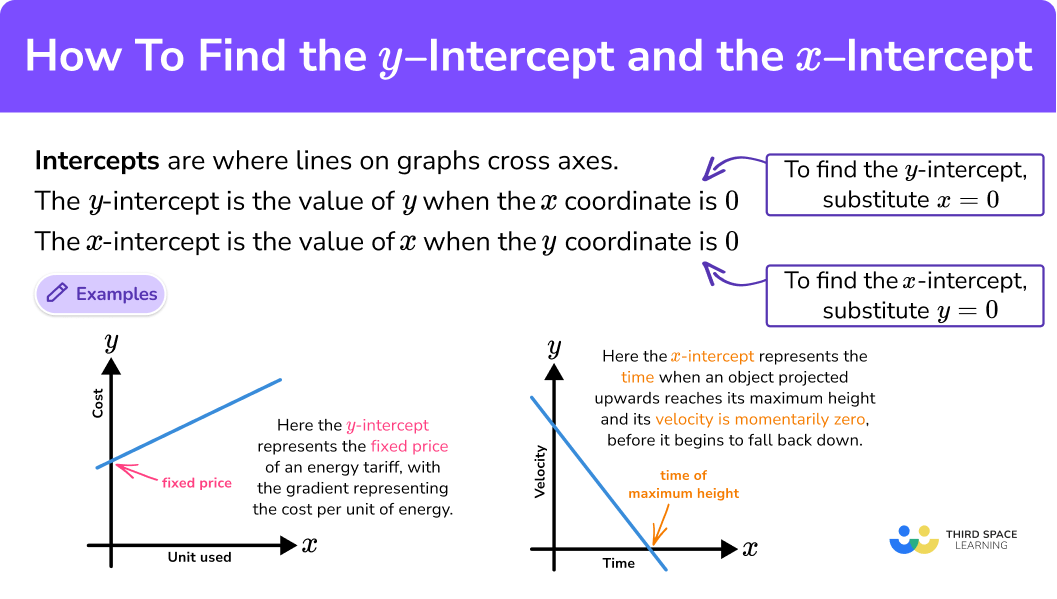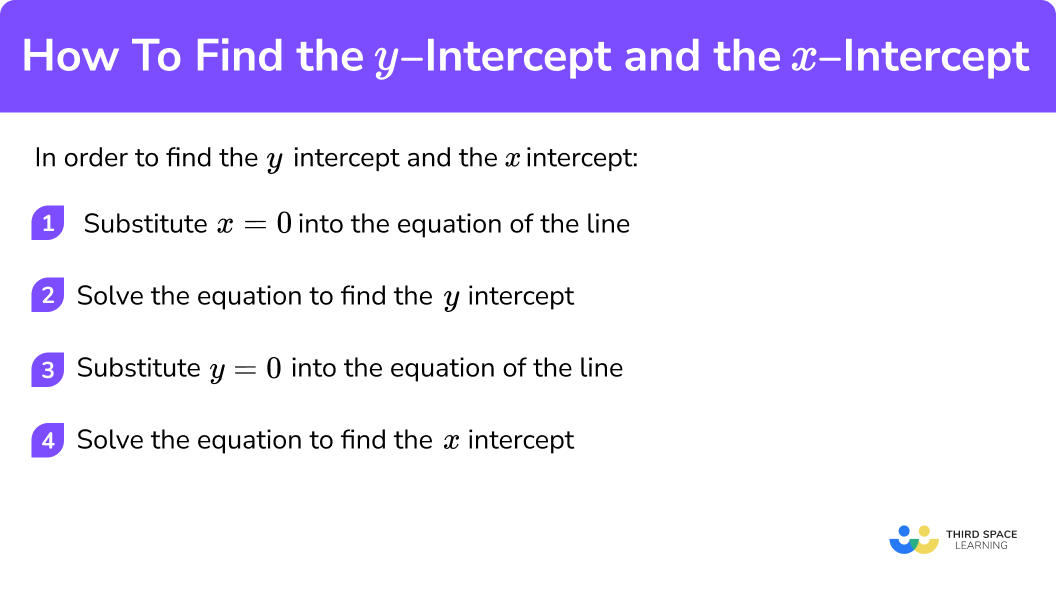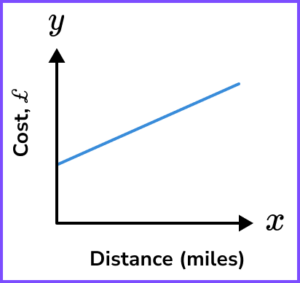# How To Find The y Intercept And The x Intercept

Here we will learn about how to find the y intercept and the x intercept from a straight line graph, including straight lines in the form y=mx+c and ax+by=c.

There are also y intercept worksheets based on Edexcel, AQA and OCR exam questions, along with further guidance on where to go next if you’re still stuck.

## What is the y intercept and the x intercept?

Finding the y intercept and the x intercept of a straight line is an important skill used to solve algebraic and real-life problems involving straight line graphs.

The intercept of a graph is where it crosses the coordinate axes

You can find the y and x intercepts of graphs of all types of functions including straight lines, quadratic functions, cubic functions and others. The x intercepts of a quadratic equation are also known as its roots. In A Level Mathematics we look at finding intercepts of more complicated functions.

To find the intercepts,

Substitute x=0 into the equation of the function to find the y intercept.

Substitute y=0 into the equation of the function to find the x intercept.

Let’s look at some examples,

A straight line has the equation 4x-3y=18. Find the y and x intercepts of the line.

• To find the y intercept, substitute x=0 into the equation.

4(0)-3y=18

Now solve the equation to find the corresponding y value.

\begin{aligned} -3y&=18\\\\ y&=-6 \end{aligned}

The y intercept is -6 and has coordinate (0,-6).

• To find the x intercept, substitute y=0 into the equation.

4x-3(0)=18

Now solve the equation to find the x value.

\begin{aligned} 4x&=18\\\\ x&=4.5 \end{aligned}

The x intercept is 4.5 and has coordinate (4.5, \ 0).

For many graphs, if the function is of the form y=[ function of x] \ + constant, the constant is the y intercept. These functions are mainly what we call polynomials, and involve integer powers of x.

For example,

However, this is not the case for more complicated functions such as reciprocals, exponentials or some trigonometric functions.

### What is the y intercept and the x intercept?## How to find the y intercept and the x intercept

In order to find the y intercept and the x intercept:

1. Substitute x=0 into the equation of the line.
2. Solve the equation to find the \bf{y} intercept.
3. Substitute y=0 into the equation of the line.
4. Solve the equation to find the \bf{x} intercept.

### Explain how to find the y intercept and the x intercept### Related lessons on straight line graphs

How to find the y intercept and the x intercept is part of our series of lessons to support revision on straight line graphs. You may find it helpful to start with the main straight line graphs lesson for a summary of what to expect, or use the step by step guides below for further detail on individual topics. Other lessons in this series include:

## How to find the y intercept and the x intercept examples

### Example 1: finding the y intercept and x intercept of a line in the form y = mx + c

Find the y intercept and x intercept of the line y=2x-5.

1. Substitute x=0 into the equation of the line.

y=2(0)-5

2Solve the equation to find the \bf{y} intercept.

This equation gives y=-5.

The y intercept is -5.

It has coordinates (0,-5).

You might have noticed that this is the special case where the equation is in the form y=[ function of x] \ + \ c. In this case c=-5 so the y intercept is (0, -5).

3Substitute y=0 into the equation of the line.

0=2x-5

4Solve the equation to find the \bf{x} intercept.

\begin{aligned} 0&=2x-5\\\\ 2x&=5\\\\ x&=2.5 \end{aligned}

The x intercept is 2.5.

It has coordinates (2.5, \ 0).

### Example 2: finding the y intercept and x intercept of a line in the form y = mx + c

Find the y intercept and x intercept of the line y=\frac{1}{2}x+3.

y=\frac{1}{2}(0)+3

This equation gives y=3.

The y intercept is 3 .

It has coordinates (0, \ 3).

0=\frac{1}{2}x+3

\begin{aligned} 0&=\frac{1}{2}x+3\\\\ \frac{1}{2}x&=-3\\\\ x&=-6 \end{aligned}

The x intercept is -6.

It has coordinates (-6, \ 0).

### Example 3: finding the y intercept and x intercept of a line in the form ax + by = c

Find the y intercept and x intercept of the line 2x+5y=20.

2(0)+5y=20

\begin{aligned} 5y&=20\\\\ y&=4 \end{aligned}

The y intercept is 4 .

It has coordinates (0, \ 4).

2x+5(0)=20

\begin{aligned} 2x&=20\\\\ x&=10 \end{aligned}

The x intercept is 10.

It has coordinates (10, \ 0).

### Example 4: finding the y intercept and x intercept of a line in the form ax + by = c

Find the y intercept and x intercept of the line 3x-4y=24.

3(0)-4y=24

\begin{aligned} -4y&=24\\\\ y&=-6 \end{aligned}

The y intercept is -6 .

It has coordinates (0, \ -6).

3x-4(0)=24

\begin{aligned} 3x&=24\\\\ x&=8 \end{aligned}

The x intercept is 8.

It has coordinates (8, \ 0).

### Example 5: finding the y intercept and x intercept of a line in context

James is measuring the height of an ice sculpture as it melts.

He thinks the height, h \ cm, can be modelled using the equation h=50-2t, where t is the number of hours after the sculpture was made.

Find the initial height of the sculpture and the time taken for it to melt completely according to James’ equation.

In this problem x is represented by t and y by h.

The initial height is the height at the start of the experiment. At the start of the experiment, no time has passed so t=0.

The initial height of the sculpture is the h intercept when t = 0.

h=50-2(0)

h=50

The h intercept is 50.

The initial height of the sculpture is 50 \ cm.

When the sculpture has melted completely, the height of the sculpture would be 0.

The time taken for the sculpture to melt completely is the t intercept when h = 0.

0=50-2t

\begin{aligned} 2t&=50\\\\ t&=25 \end{aligned}

The t intercept is 25.

It takes 25 hours for the sculpture to melt completely.

### Common misconceptions

• Substituting the wrong value for zero

A common error is to think that the y intercept is when y = 0.

It is important to remember that when y = 0, the line will be crossing the x axis and therefore will give the x intercept.

• Forgetting to state the coordinates when asked

If an exam question asks for the y intercept or x intercept, just the value where the line crosses the axes is appropriate. However, sometimes the question will ask for the coordinates of the y intercept. In this case the answer must be given in the form (0, \ c), or (a, \ 0) for the coordinates of the x intercept.

• Confusing the intercept with the gradient

When the line is in the form y=mx+c, a common error is to confuse the y intercept, c with the gradient or slope of a line, m. The y intercept will have coordinates (0, \ c) and the x intercept will have coordinates (-\frac{c}{m}, \ 0).

### Practice how to find the y intercept and the x intercept questions

1. State the coordinate of the y intercept of the line y=3x-2.

(-2,0)(0,-2)(3,0)(0,3)The coordinate of the y intercept of a line in the form y=mx+c will be (0,c).

2. Find the coordinate of the x intercept of the line y=3x-6.

(2,0)(0,2)(-2,0)(-6,0)Substitute y=0 to give

\begin{aligned} 0&=3x-6\\\\ 3x&=6 \\\\ x&=2 \end{aligned}

3. The equation of a line is given as y=8-3x.

Find the y intercept and x intercept of the line.

y intercept = \frac{8}{3}, \ x intercept = 8y intercept = 8, \ x intercept = -3y intercept = 8, \ x intercept = -\frac{8}{3}y intercept = 8, \ x intercept = \frac{8}{3}When x=0, \ y=8-3(0)=8

When y=0,

\begin{aligned} 0&=8-3x\\\\ 3x&=8 \\\\ x&=\frac{8}{3} \end{aligned}

4. The equation of a line is given as x-3y=9.

Find the y intercept and x intercept of the line.

y intercept = -3, \ x intercept = 9y intercept = 9, \ x intercept = -3y intercept = -\frac{1}{3}, \ x intercept = 9y intercept = 9, \ x intercept = -\frac{1}{3}When x=0,

\begin{aligned} -3y&=9\\\\ y&=-3 \end{aligned}

When y=0,

\begin{aligned} x-3(0)&=9\\\\ x&=9 \end{aligned}

5. The equation of a line is given as 5x-4y=10.

Find the y intercept and x intercept of the line.

y intercept = 2, \ x intercept = -2.5y intercept = -2.5, \ x intercept = 2y intercept = -\frac{2}{5}, \ x intercept = \frac{1}{2}y intercept = \frac{1}{2}, \ x intercept = -\frac{2}{5}When x=0,

\begin{aligned} 5(0)-4y&=10\\\\ -4y&=10\\\\ y&=-2.5 \end{aligned}

When y=0,

\begin{aligned} 5x-4(0)&=10\\\\ 5x&=10\\\\ x&=2 \end{aligned}

6. An object is projected vertically upwards and its velocity, v, is modelled by the equation v=14-10t, where t is the time in seconds after its initial projection.

Find the time when the object reaches its maximum height.

1.4 seconds14 seconds10 seconds0.71 secondsMaximum height occurs when v = 0, which is the t intercept of the line equation.

\begin{aligned} 0&=14-10t\\\\ 10t&=14\\\\ t&=1.4 \end{aligned}

### How to find the y intercept and the x intercept GCSE questions

1. The cost £C of hiring a taxi for a journey of m miles is given by the formula,

C=0.6m+5.

The cost is plotted on the following set of axes.(a) State the y intercept of the line.

(b) Give an interpretation of the y intercept.

(2 marks)

(a) 5

(1)

(b) The price of hiring the taxi before it has travelled any distance / a fixed cost added to the price of each journey.

(1)

2. A line has the equation 6x-3y=12.

(a) Find the y intercept of the line.

(b) Find the x intercept of the line.

(4 marks)

(a)

Substituting x=0.

(1)

Solving to give y = – 4.

(1)

(b)

Substituting y=0.

(1)

Solving to give 2.

(1)

3. The line L passes through the point (3,5) and has gradient 2.

The line crosses the x axis at point A.

Write down the coordinates of point A.

(4 marks)

Method using the point (3,5) and gradient of 2 to find the equation.

(1)

Equation of y=2x-1 found.

(1)

Substituting y=0.

(1)

Solving to give x=\frac{1}{2} \ \text{or} \ (\frac{1}{2},0).

(1)

## Learning checklist

You have now learned how to:

• Find the y intercept and x intercept of straight line graphs

## Still stuck?

Prepare your KS4 students for maths GCSEs success with Third Space Learning. Weekly online one to one GCSE maths revision lessons delivered by expert maths tutors.

Find out more about our GCSE maths tuition programme.